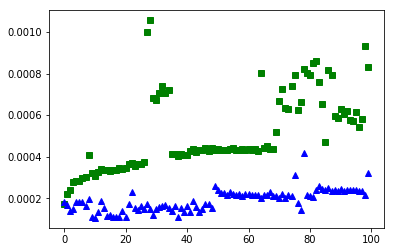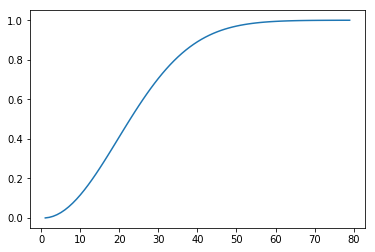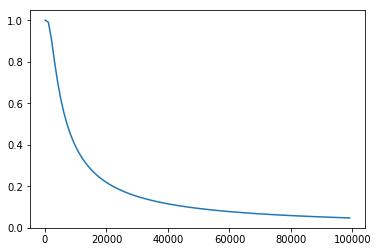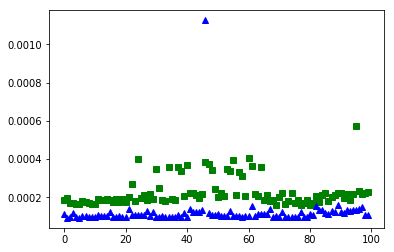# Hashtables¶

## Agenda¶

• Discussion: pros/cons of array-backed and linked structures
• Python's other built-in DS: the dict
• A naive lookup DS
• Direct lookups via Hashing
• Hashtables
• Collisions and the "Birthday problem"
• Runtime analysis & Discussion

## Discussion: pros/cons of array-backed and linked structures¶

Between the array-backed and linked list we have:

1. $O(1)$ indexing (array-backed)
2. $O(1)$ appending (array-backed & linked)
3. $O(1)$ insertion/deletion without indexing (linked)
4. $O(\log N)$ binary search, when sorted (array-backed)

## Python's other built-in DS: the dict¶

In :
# by default, only the result of the last expression in a cell is displayed after evaluation.
# the following forces display of *all* self-standing expressions in a cell.

from IPython.core.interactiveshell import InteractiveShell
InteractiveShell.ast_node_interactivity = "all"

In :
import timeit

def lin_search(lst, x):
for i in range(len(lst)):
if lst[i] == x:
return i
raise ValueError(x)

def bin_search(lst, x):
# assume that lst is sorted!!!
low = 0
hi  = len(lst)
mid = (low + hi) // 2
while lst[mid] != x and low <= hi:
if lst[mid] < x:
low = mid + 1
else:
hi  = mid - 1
mid = (low + hi) // 2
if lst[mid] == x:
return mid
else:
raise ValueError(x)

def time_lin_search(size):
return timeit.timeit('lin_search(lst, random.randrange({}))'.format(size), # interpolate size into randrange
'import random ; from __main__ import lin_search ;'
'lst = [x for x in range({})]'.format(size), # interpolate size into list range
number=100)

def time_bin_search(size):
return timeit.timeit('bin_search(lst, random.randrange({}))'.format(size), # interpolate size into randrange
'import random ; from __main__ import bin_search ;'
'lst = [x for x in range({})]'.format(size), # interpolate size into list range
number=100)

def time_dict(size):
return timeit.timeit('dct[random.randrange({})]'.format(size),
'import random ; '
'dct = {{x: x for x in range({})}}'.format(size),
number=100)

lin_search_timings = [time_lin_search(n)
for n in range(10, 10000, 100)]

bin_search_timings = [time_bin_search(n)
for n in range(10, 10000, 100)]

dict_timings = [time_dict(n)
for n in range(10, 10000, 100)]

In :
%matplotlib inline
import matplotlib.pyplot as plt
#plt.plot(lin_search_timings, 'ro')
plt.plot(bin_search_timings, 'gs')
plt.plot(dict_timings, 'b^')
plt.show()## A naive lookup DS
In :
class LookupDS:
def __init__(self):
self.data = []

def __setitem__(self, key, value):
# look for the key, update the value, or add the key/value
for i in range(len(self.data)):
if self.data[i] == key:
self.data[i] = value
break
else:
self.data.append([key, value])

def __getitem__(self, key):

#  find the key and return the value, or raise KeyValue exception
#      for i in range(len(self.data)):
#          if self.data[i] == key:
#              return self.data[i]
#      else:
#          raise KeyError()
#pythonic
for item in self.data:
if item==key:
return item
else:
raise KeyError()

def __contains__(self, key):

#  find the key return true or false
try:
_ = self[key]
return True
except:
return False


In :
l = LookupDS()
l['batman'] = 'bruce wayne'
l['superman'] = 'clark kent'
l['spiderman'] = 'peter parker'
l['batman']

Out:
'bruce wayne'

## Direct lookups via Hashing¶

Hashes (a.k.a. hash codes or hash values) are simply numerical values computed for objects.

In :
hash('hello')

Out:
4567778509397514502
In :
hash('batman')

Out:
-5039084676607491960
In :
hash('batmen')

Out:
-7208566709597815098
In :
[hash(s) for s in ['different', 'objects', 'have', 'very', 'different', 'hashes']]

Out:
[4900375171937521485,
-8746326281165982360,
-8921093800637269788,
-5875884575928133559,
4900375171937521485,
-2018829733429993967]
In [ ]:
[i%100 for i in range(10, 1000, 40)]

In :
[hash(s)%100 for s in ['different', 'objects', 'have', 'very', 'different', 'hashes']]

Out:
[85, 40, 12, 41, 85, 33]

## Hashtables¶

In :
class Hashtable:
def __init__(self, n_buckets=1000):
self.buckets = [None] * n_buckets

def __setitem__(self, key, val):
# hash the key and mod to get an index, store the value using []
bucket_idx = hash(key) % len(self.buckets)
self.buckets[bucket_idx] = val

def __getitem__(self, key):
# hash the key and mod to get an index, if exist return the value else raise KeyError
bucket_idx = hash(key) % len(self.buckets)
if self.buckets[bucket_idx]:
return self.buckets[bucket_idx]
else:
raise KeyError

def __contains__(self, key):
try:
_ = self[key]
return True
except:
return False

In :
ht = Hashtable(10)
ht['batman'] = 'bruce wayne'
ht['superman'] = 'clark kent'
ht['spiderman'] = 'peter parker'
ht['superman']
ht['antman']

Out:
'clark kent'
---------------------------------------------------------------------------
KeyError                                  Traceback (most recent call last)
<ipython-input-6-aaf92435763d> in <module>()
4 ht['spiderman'] = 'peter parker'
5 ht['superman']
----> 6 ht['antman']

<ipython-input-3-103c38188216> in __getitem__(self, key)
15             return self.buckets[bucket_idx]
16         else:
---> 17             raise KeyError
18
19

KeyError: 

## On Collisions¶

### The "Birthday Problem"¶

Problem statement: Given $N$ people at a party, how likely is it that at least two people will have the same birthday?

In :
def birthday_p(n_people):
p_inv = 1
for n in range(365, 365-n_people, -1):
p_inv *= n / 365
return 1 - p_inv

In :
birthday_p(2)

Out:
0.002739726027397249
In :
1-364/365

Out:
0.002739726027397249
In :
%matplotlib inline
import matplotlib.pyplot as plt

n_people = range(1, 80)
plt.plot(n_people, [birthday_p(n) for n in n_people])
plt.show()

Out:
[<matplotlib.lines.Line2D at 0x8d8b780>]### General collision statistics¶

Repeat the birthday problem, but with a given number of values and "buckets" that are allotted to hold them. How likely is it that two or more values will map to the same bucket?

In :
def collision_p(n_values, n_buckets):
p_inv = 1
for n in range(n_buckets, n_buckets-n_values, -1):
p_inv *= n / n_buckets
return 1 - p_inv

In :
collision_p(23, 365) # same as birthday problem, for 23 people

Out:
0.5072972343239857
In :
collision_p(10, 100)

Out:
0.37184349044470544
In :
collision_p(100, 1000)

Out:
0.9940410733677595
In :
# keeping number of values fixed at 100, but vary number of buckets: visualize probability of collision
%matplotlib inline
import matplotlib.pyplot as plt

n_buckets = range(100, 100001, 1000)
plt.plot(n_buckets, [collision_p(100, nb) for nb in n_buckets])
plt.show()

Out:
[<matplotlib.lines.Line2D at 0x9282160>]In :
def avg_num_collisions(n, b):
"""Returns the expected number of collisions for n values uniformly distributed
over a hashtable of b buckets. Based on (fairly) elementary probability theory.
(Pay attention in MATH 474!)"""
return n - b + b * (1 - 1/b)**n

In :
avg_num_collisions(28, 365)

Out:
1.011442040700615
In :
avg_num_collisions(1000, 1000)

Out:
367.6954247709637
In :
avg_num_collisions(1000, 10000)

Out:
48.32893558556316

## Dealing with Collisions¶

To deal with collisions in a hashtable, we simply create a "chain" of key/value pairs for each bucket where collisions occur. The chain needs to be a data structure that supports quick insertion — natural choice: the linked list!

In :
class Hashtable:
class Node:
def __init__(self, key, val, next=None):
self.key = key
self.val = val
self.next = next

def __init__(self, n_buckets=1000):
self.buckets = [None] * n_buckets

def __setitem__(self, key, val):
# get the node at the bucket_idx [] location, while not none, walk the list looking for the key, if found set the value, else insert a new node at start of the list
bucket_idx = hash(key) % len(self.buckets)
n = self.buckets[bucket_idx]
while n:
if n.key == key:
n.val = val
return
n = n.next
else:
self.buckets[bucket_idx] = Hashtable.Node(key, val, self.buckets[bucket_idx])

def __getitem__(self, key):
# get the node at the bucket_idx [] location, while not none, walk the list looking for the key, if found return the value, else raise KeyError
bucket_idx = hash(key) % len(self.buckets)
n = self.buckets[bucket_idx]
while n:
if n.key == key:
return n.val
n = n.next
raise KeyError

def __contains__(self, key):
try:
_ = self[key]
return True
except:
return False

In :
ht = Hashtable(1)
ht['batman'] = 'bruce wayne'
ht['superman'] = 'clark kent'
ht['spiderman'] = 'peter parker'
ht['spiderman']
ht['batman']

Out:
'peter parker'
Out:
'bruce wayne'
In :
def prep_ht(size):
ht = Hashtable(size*10)
for x in range(size):
ht[x] = x
return ht

def time_ht(size):
return timeit.timeit('ht[random.randrange({})]'.format(size),
'import random ; from __main__ import prep_ht ;'
'ht = prep_ht({})'.format(size),
number=100)

ht_timings = [time_ht(n)
for n in range(10, 10000, 100)]

In :
%matplotlib inline
import matplotlib.pyplot as plt
#plt.plot(bin_search_timings, 'ro')
plt.plot(ht_timings, 'gs')
plt.plot(dict_timings, 'b^')
plt.show()

Out:
[<matplotlib.lines.Line2D at 0x92e6a20>]
Out:
[<matplotlib.lines.Line2D at 0x92d75f8>]## Loose ends¶

### Iteration¶

In :
class Hashtable(Hashtable):
def __iter__(self):
for item in self.buckets:
while item != None:
yield item.key
item=item.next

In :
ht = Hashtable(100)
ht['batman'] = 'bruce wayne'
ht['superman'] = 'clark kent'
ht['spiderman'] = 'peter parker'

In :
for k in ht:
print(k)

superman
batman
spiderman


### Key ordering¶

In :
ht = Hashtable()
d = {}
for x in 'apple banana cat dog elephant'.split():
d[x] = x
ht[x] = x

In :
for k in d:
print(k, '=>', d[k])

a => apple
b => banana
c => cat
d => dog
e => elephant

In :
for k in ht:
print(k, '=>', ht[k])

c => cat
e => elephant
a => apple
b => banana
d => dog


It doesn't often make sense to start with a large number of buckets, unless we know in advance that the number of keys is going to be vast — also, the user of the hashtable would typically prefer to not be bothered with implementation details (i.e., bucket count) when using the data structure.

Instead: start with a relatively small number of buckets, and if the ratio of keys to the number of buckets (known as the load factor) is above some desired threshold — which we can determine using collision probabilities — we can dynamically increase the number of buckets. This requires, however, that we rehash all keys and potentially move them into new buckets (since the hash(key) % num_buckets mapping will likely be different with more buckets).

### Other APIs¶

• FIXED __setitem__ (to update value for existing key)
• __delitem__
• keys & values (return iterators for keys and values)
• setdefault

## Runtime analysis & Discussion¶

For a hashtable with $N$ key/value entries:

• Insertion: $O(?)$
• Lookup: $O(?)$
• Deletion: $O(?)$

## Vocabulary list¶

• hashtable
• hashing and hashes
• collision
• hash buckets & chains
• birthday problem
• rehashing

Remember: a given object must always hash to the same value. This is required so that we can always map the object to the same hash bucket.

Hashcodes for collections of objects are usually computed from the hashcodes of its contents, e.g., the hash of a tuple is a function of the hashes of the objects in said tuple:

In [ ]:
hash(('two', 'strings'))


This is useful. It allows us to use a tuple, for instance, as a key for a hashtable.

However, if the collection of objects is mutable — i.e., we can alter its contents — this means that we can potentially change its hashcode.

If we were to use such a collection as a key in a hashtable, and alter the collection after it's been assigned to a particular bucket, this leads to a serious problem: the collection may now be in the wrong bucket (as it was assigned to a bucket based on its original hashcode)!

For this reason, only immutable types are, by default, hashable in Python. So while we can use integers, strings, and tuples as keys in dictionaries, lists (which are mutable) cannot be used. Indeed, Python marks built-in mutable types as "unhashable", e.g.,

In [ ]:
hash([1, 2, 3])


That said, Python does support hashing on instances of custom classes (which are mutable). This is because the default hash function implementation does not rely on the contents of instances of custom classes. E.g.,

In [ ]:
class Student:
def __init__(self, fname, lname):
self.fname = fname
self.lname = lname

In [ ]:
s = Student('John', 'Doe')
hash(s)

In [ ]:
s.fname = 'Jane'
hash(s) # same as before mutation


We can change the default behavior by providing our own hash function in __hash__, e.g.,

In [ ]:
class Student:
def __init__(self, fname, lname):
self.fname = fname
self.lname = lname

def __hash__(self):
return hash(self.fname) + hash(self.lname)

In [ ]:
s = Student('John', 'Doe')
hash(s)

In [ ]:
s.fname = 'Jane'
hash(s)
`

But be careful: instances of this class are no longer suitable for use as keys in hashtables (or dictionaries), if you intend to mutate them after using them as keys!